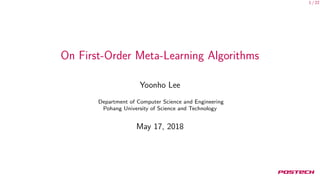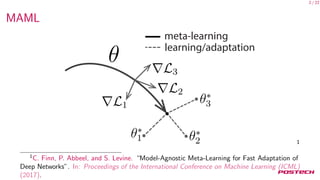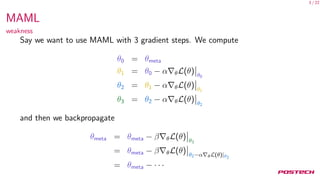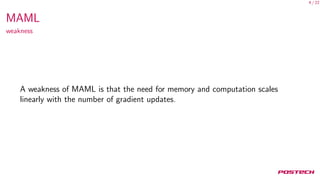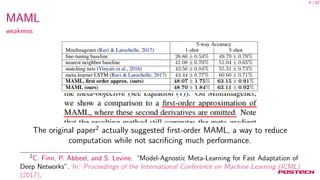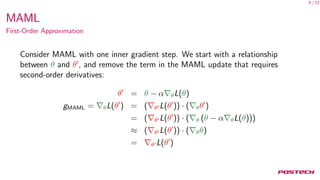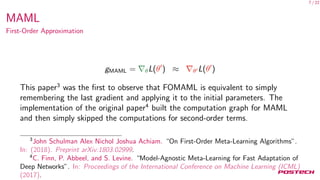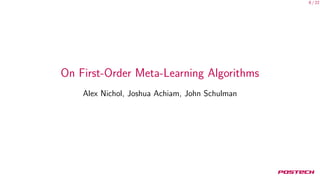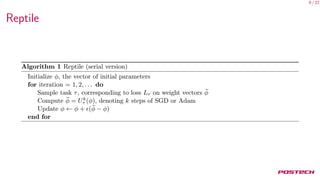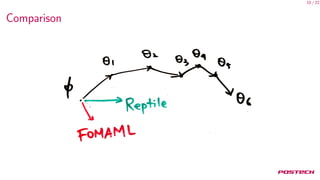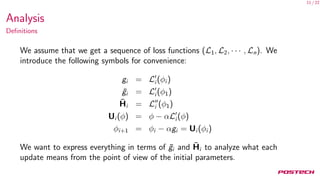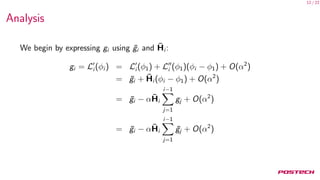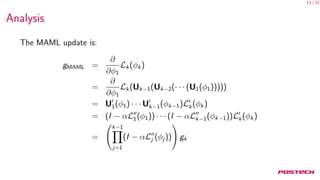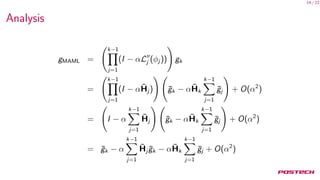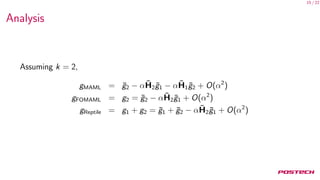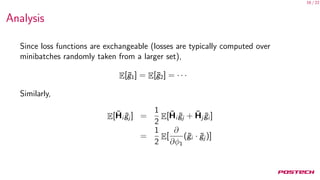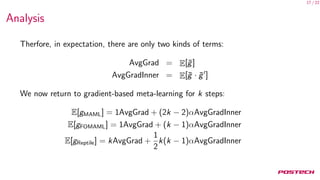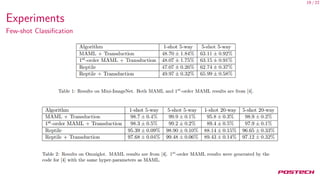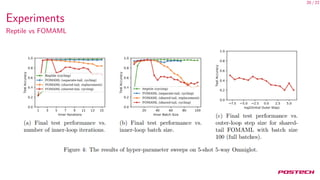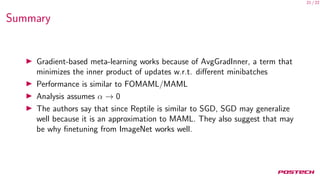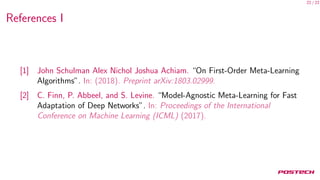1 of 23

### On First-Order Meta-Learning Algorithms

1. 1 / 22 On First-Order Meta-Learning Algorithms Yoonho Lee Department of Computer Science and Engineering Pohang University of Science and Technology May 17, 2018
2. 2 / 22 MAML 1 1 C. Finn, P. Abbeel, and S. Levine. “Model-Agnostic Meta-Learning for Fast Adaptation of Deep Networks”. In: Proceedings of the International Conference on Machine Learning (ICML) (2017).
3. 3 / 22 MAML weakness Say we want to use MAML with 3 gradient steps. We compute θ0 = θmeta θ1 = θ0 − α θL(θ) θ0 θ2 = θ1 − α θL(θ) θ1 θ3 = θ2 − α θL(θ) θ2 and then we backpropagate θmeta = θmeta − β θL(θ) θ3 = θmeta − β θL(θ) θ2−α θL(θ)|θ2 = θmeta − · · ·
4. 4 / 22 MAML weakness A weakness of MAML is that the need for memory and computation scales linearly with the number of gradient updates.
5. 5 / 22 MAML weakness The original paper2 actually suggested ﬁrst-order MAML, a way to reduce computation while not sacriﬁcing much performance. 2 C. Finn, P. Abbeel, and S. Levine. “Model-Agnostic Meta-Learning for Fast Adaptation of Deep Networks”. In: Proceedings of the International Conference on Machine Learning (ICML) (2017).
6. 6 / 22 MAML First-Order Approximation Consider MAML with one inner gradient step. We start with a relationship between θ and θ , and remove the term in the MAML update that requires second-order derivatives: θ = θ − α θL(θ) gMAML = θL(θ ) = ( θ L(θ )) · ( θθ ) = ( θ L(θ )) · ( θ (θ − α θL(θ))) ≈ ( θ L(θ )) · ( θθ) = θ L(θ )
7. 7 / 22 MAML First-Order Approximation gMAML = θL(θ ) ≈ θ L(θ ) This paper3 was the ﬁrst to observe that FOMAML is equivalent to simply remembering the last gradient and applying it to the initial parameters. The implementation of the original paper4 built the computation graph for MAML and then simply skipped the computations for second-order terms. 3 John Schulman Alex Nichol Joshua Achiam. “On First-Order Meta-Learning Algorithms”. In: (2018). Preprint arXiv:1803.02999. 4 C. Finn, P. Abbeel, and S. Levine. “Model-Agnostic Meta-Learning for Fast Adaptation of Deep Networks”. In: Proceedings of the International Conference on Machine Learning (ICML) (2017).
8. 8 / 22 On First-Order Meta-Learning Algorithms Alex Nichol, Joshua Achiam, John Schulman
9. 9 / 22 Reptile
10. 10 / 22 Comparison
11. 11 / 22 Analysis Deﬁnitions We assume that we get a sequence of loss functions (L1, L2, · · · , Ln). We introduce the following symbols for convenience: gi = Li (φi ) ¯gi = Li (φ1) ¯Hi = Li (φ1) Ui (φ) = φ − αLi (φ) φi+1 = φi − αgi = Ui (φi ) We want to express everything in terms of ¯gi and ¯Hi to analyze what each update means from the point of view of the initial parameters.
12. 12 / 22 Analysis We begin by expressing gi using ¯gi and ¯Hi : gi = Li (φi ) = Li (φ1) + Li (φ1)(φi − φ1) + O(α2 ) = ¯gi + ¯Hi (φi − φ1) + O(α2 ) = ¯gi − α ¯Hi i−1 j=1 gj + O(α2 ) = ¯gi − α ¯Hi i−1 j=1 ¯gj + O(α2 )
13. 13 / 22 Analysis The MAML update is: gMAML = ∂ ∂φ1 Lk(φk) = ∂ ∂φ1 Lk(Uk−1(Uk−2(· · · (U1(φ1))))) = U1(φ1) · · · Uk−1(φk−1)Lk(φk) = (I − αL1(φ1)) · · · (I − αLk−1(φk−1))Lk(φk) = k−1 j=1 (I − αLj (φj )) gk
14. 14 / 22 Analysis gMAML = k−1 j=1 (I − αLj (φj )) gk = k−1 j=1 (I − α ¯Hj ) ¯gk − α ¯Hk k−1 j=1 ¯gj + O(α2 ) = I − α k−1 j=1 ¯Hj ¯gk − α ¯Hk k−1 j=1 ¯gj + O(α2 ) = ¯gk − α k−1 j=1 ¯Hj ¯gk − α ¯Hk k−1 j=1 ¯gj + O(α2 )
15. 15 / 22 Analysis Assuming k = 2, gMAML = ¯g2 − α ¯H2 ¯g1 − α ¯H1 ¯g2 + O(α2 ) gFOMAML = g2 = ¯g2 − α ¯H2 ¯g1 + O(α2 ) gReptile = g1 + g2 = ¯g1 + ¯g2 − α ¯H2 ¯g1 + O(α2 )
16. 16 / 22 Analysis Since loss functions are exchangeable (losses are typically computed over minibatches randomly taken from a larger set), E[¯g1] = E[¯g2] = · · · Similarly, E[ ¯Hi ¯gj ] = 1 2 E[ ¯Hi ¯gj + ¯Hj ¯gi ] = 1 2 E[ ∂ ∂φ1 (¯gi · ¯gj )]